从零开始造一个“智障”聊天机器人

0x1 基本概念

01｜神经网络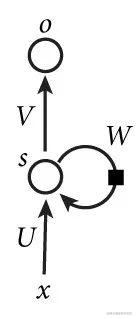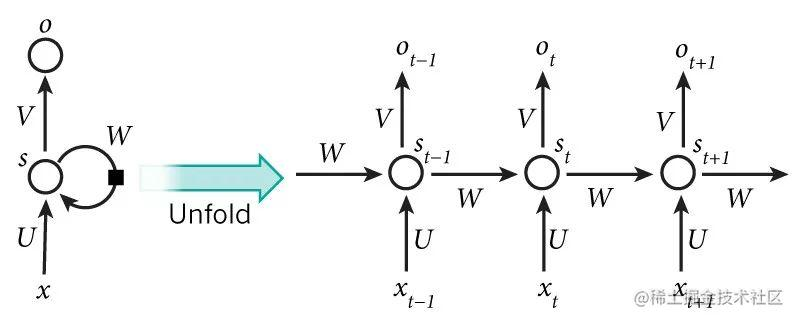02｜深度学习框架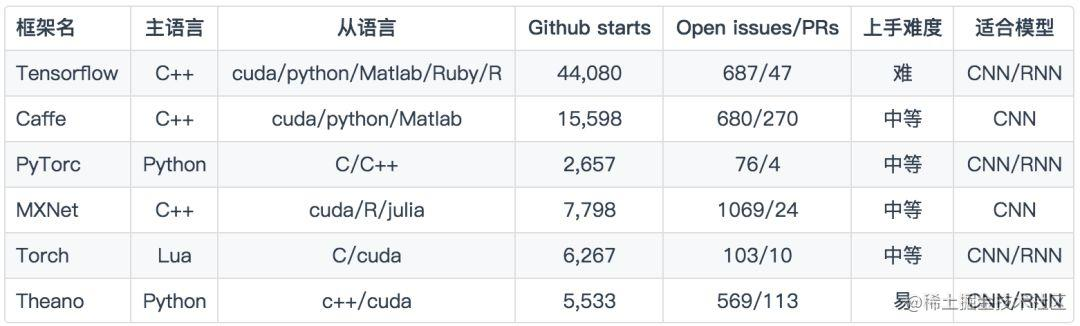03｜seq2seq模型

Step1应用双向最大匹配算法分词：双向分词结果，正向《大白天，的，做什么，美梦，啊》；反向《大白天，的，做什么，美梦，啊》。正向反向都是一样的，所以不需要处理歧义问题。长词优先选择，“大白天”和“做什么”。

Step2：以“大白天”举例，假设hash函数为f（），并设f（大白天）指向首字hash表项[大，11，P]。于是由该表项指向“3字索引”，再指向对应“词表”。

Step3将结构体<大白天，…>插入队尾。体中有一个Ans域，域中某一指针指向“哦哈哈哈不用你管”。

0x2 语料准备

01｜语料整理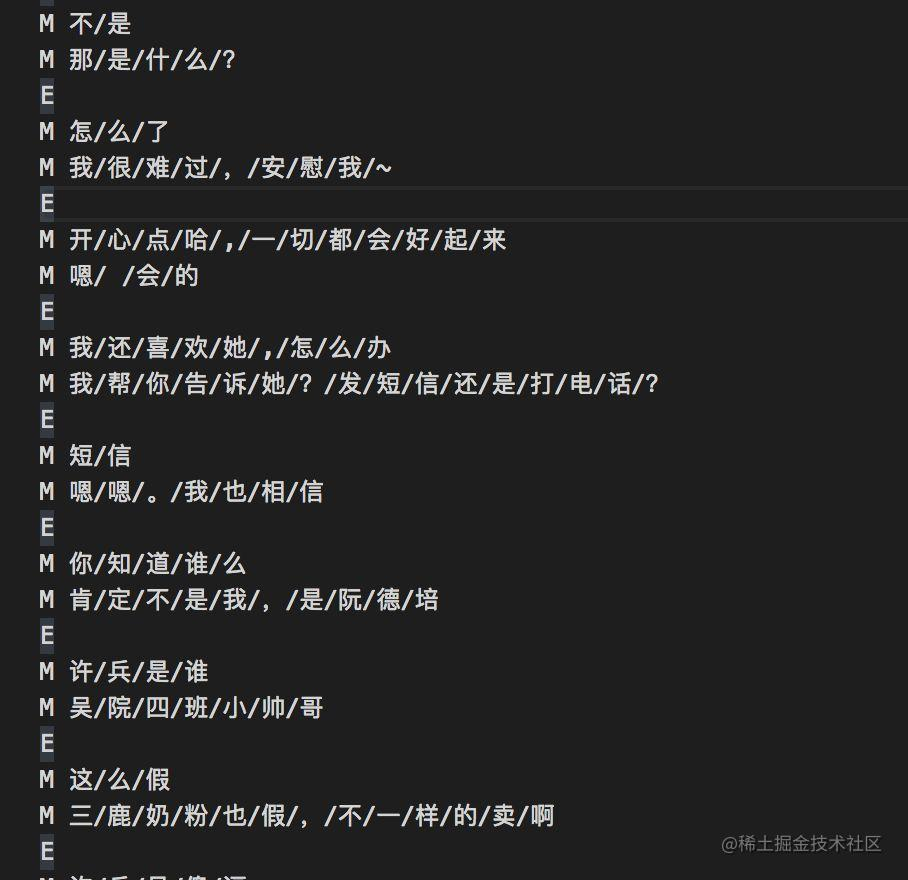1import re
2import sys
3def prepare(num_dialogs=50000):
4    with open("xhj.conv"as fopen:
5        # 替换E、M等
6        reg = re.compile("EnM (.*?)nM (.*?)n")
8        # 使用5W条对话作为训练语料
9        if num_dialogs >= len(match_dialogs):
10            dialogs = match_dialogs
11        else:
12            dialogs = match_dialogs[:num_dialogs]
13        questions = []
15        for que, ans in dialogs:
16            questions.append(que)
18        # 保存到data/文件夹目录下
19        save(questions, "data/Question.txt")
21def save(dialogs, file):
22    with open(file, "w"as fopen:
23        fopen.write("n".join(dialogs))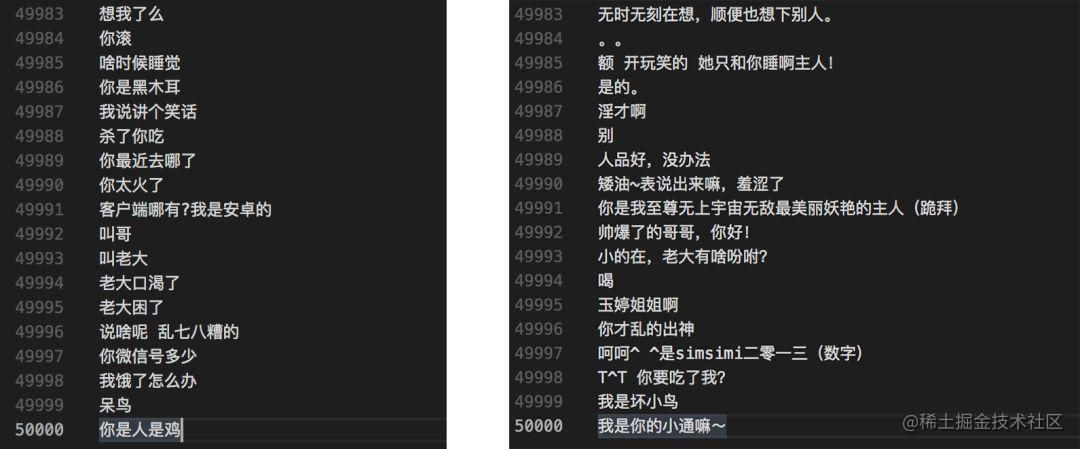02｜向量表映射建立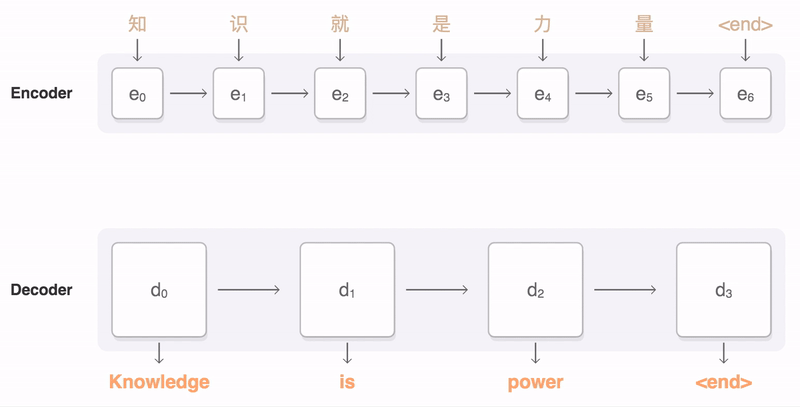1def gen_vocabulary_file(input_file, output_file):
2    vocabulary = {}
3    with open(input_file) as f:
4        counter = 0
5        for line in f:
6            counter += 1
7            tokens = [word for word in line.strip()]
8            for word in tokens:
9                                # 过滤非中文 文字
10                if u'u4e00' <= word <= u'u9fff':
11                    if word in vocabulary:
12                        vocabulary[word] += 1
13                    else:
14                        vocabulary[word] = 1
15        vocabulary_list = START_VOCABULART + sorted(vocabulary, key=vocabulary.get, reverse=True)
16        # 取前3500个常用汉字，vocabulary_size = 3500
17        if len(vocabulary_list) > vocabulary_size:
18            vocabulary_list = vocabulary_list[:vocabulary_size]
19        print(input_file + " 词汇表大小:", len(vocabulary_list))
20        with open(output_file, "w"as ff:
21            for word in vocabulary_list:
22                ff.write(word + "n")
23        ff.close

0x3 开始训练

01｜训练

1# 源输入词表的大小
2vocabulary_encode_size = 3500
3# 目标输出词表的大小
4vocabulary_decode_size = 3500
5#一种有效处理不同长度的句子的方法
6buckets = [(510), (1015), (2025), (4050)]
7# 每层单元数目
8layer_size = 256
9# 网络的层数。
10num_layers = 3
11# 训练时的批处理大小
12batch_size =  64
14# learning_rate： 初始的学习率
15# learning_rate_decay_factor： 学习率衰减因子
16# forward_only： false意味着在解码器端，使用decoder_inputs作为输入。例如decoder_inputs 是‘GO, W, X, Y, Z ’，正确的输出应该是’W, X, Y, Z, EOS’。假设第一个时刻的输出不是’W’，在第二个时刻也要使用’W’作为输入。当设为true时，只使用decoder_inputs的第一个时刻的输入，即’GO’，以及解码器的在每一时刻的真实输出作为下一时刻的输入。
17model = seq2seq_model.Seq2SeqModel(source_vocab_size=vocabulary_encode_size, target_vocab_size=vocabulary_decode_size,buckets=buckets, size=layer_size, num_layers=num_layers, max_gradient_norm= 5.0,batch_size=batch_size, learning_rate=0.5, learning_rate_decay_factor=0.97, forward_only=False)
18
19config = tf.ConfigProto()
20config.gpu_options.allocator_type = 'BFC'  # 防止 out of memory
21
22with tf.Session(config=config) as sess:
23    # 恢复前一次训练
24    ckpt = tf.train.get_checkpoint_state('.')
25    if ckpt != None:
26        print(ckpt.model_checkpoint_path)
27        model.saver.restore(sess, ckpt.model_checkpoint_path)
28    else:
29        sess.run(tf.global_variables_initializer())
30
33
34    train_bucket_sizes = [len(train_set[b]) for b in range(len(buckets))]
35    train_total_size = float(sum(train_bucket_sizes))
36    train_buckets_scale = [sum(train_bucket_sizes[:i + 1]) / train_total_size for i in range(len(train_bucket_sizes))]
37
38    loss = 0.0
39    total_step = 0
40    previous_losses = []
41    # 一直训练，每过一段时间保存一次模型
42    while True:
43        random_number_01 = np.random.random_sample()
44        bucket_id = min([i for i in range(len(train_buckets_scale)) if train_buckets_scale[i] > random_number_01])
45
46        encoder_inputs, decoder_inputs, target_weights = model.get_batch(train_set, bucket_id)
47        _, step_loss, _ = model.step(sess, encoder_inputs, decoder_inputs, target_weights, bucket_id, False)
48
49        loss += step_loss / 500
50        total_step += 1
51
52        print(total_step)
53        if total_step % 500 == 0:
54            print(model.global_step.eval(), model.learning_rate.eval(), loss)
55
56            # 如果模型没有得到提升，减小learning rate
57            if len(previous_losses) > 2 and loss > max(previous_losses[-3:]):
58                sess.run(model.learning_rate_decay_op)
59            previous_losses.append(loss)
60            # 保存模型
61            checkpoint_path = "chatbot_seq2seq.ckpt"
62            model.saver.save(sess, checkpoint_path, global_step=model.global_step)
63            loss = 0.0
64            # 使用测试数据评估模型
65            for bucket_id in range(len(buckets)):
66                if len(test_set[bucket_id]) == 0:
67                    continue
68                encoder_inputs, decoder_inputs, target_weights = model.get_batch(test_set, bucket_id)
69                _, eval_loss, _ = model.step(sess, encoder_inputs, decoder_inputs, target_weights, bucket_id, True)
70                eval_ppx = math.exp(eval_loss) if eval_loss < 300 else float('inf')
71                print(bucket_id, eval_ppx)

02｜实际问答效果

① Perplexity是什么：

PPL是用在自然语言处理领域（NLP）中，衡量语言模型好坏的指标。它主要是根据每个词来估计一句话出现的概率，并用句子长度作normalize，公式为 :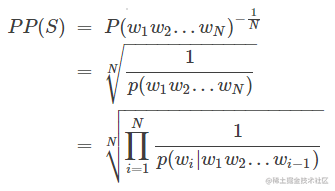S代表sentence，N是句子长度，p(wi)是第i个词的概率。第一个词就是 p(w1|w0)，而w0是START，表示句子的起始，是个占位符。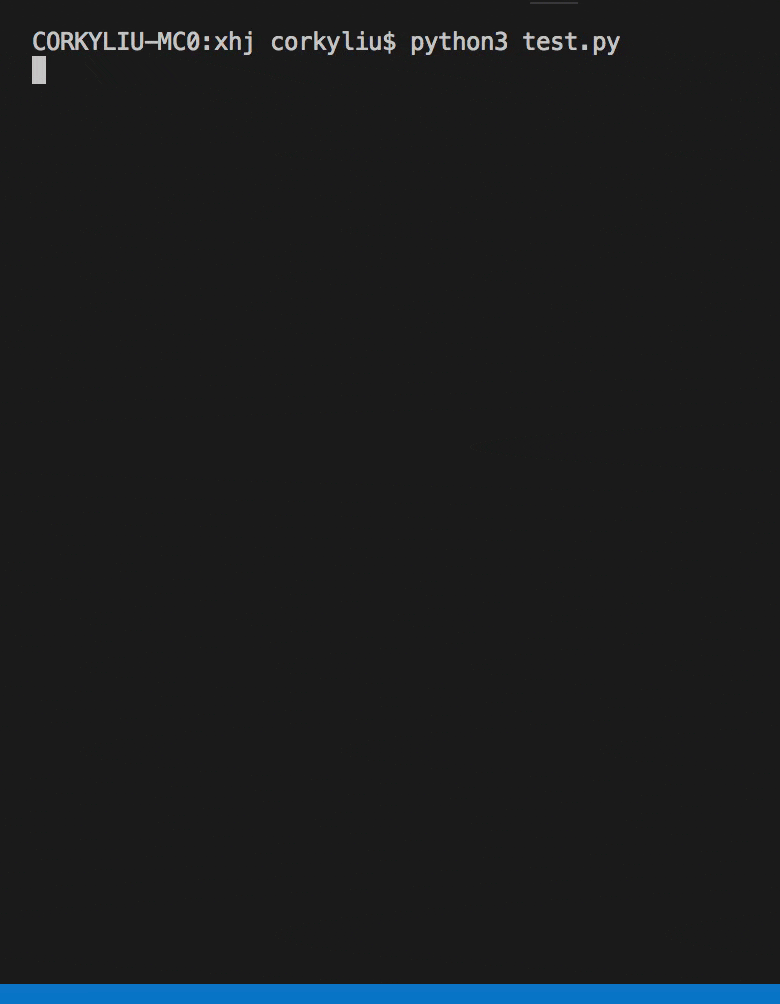0x4 结语